﻿ 结合跳点引导的无人机随机搜索避撞决策方法Download PDF文章快速检索 高级检索

1. 中国民用航空飞行学院 空中交通管理学院, 广汉 618307;
2. 乔治·华盛顿大学 机械与航空航天工程学院, 华盛顿特区 20052

Collision avoidance decision method for UAVs in random search combined with jump point guidance
LI Anti1, LI Chenglong1, WU Dingjie1, WEI Peng2
1. College of Air Traffic Management, Civil Aviation Flight University of China, Guanghan 618307, China;
2. Department of Mechanical and Aerospace Engineering, George Washington University, Washington, D. C. 20052, USA
Abstract: Aiming at the collision avoidance decision of UAVs in urban airspace environment and dense air traffic flow, Markov Decision Process (MDP) and Monte Carlo Tree Search (MCTS) algorithms are proposed to model and solve this problem. To ensure real-time performance, the MCTS algorithm is limited in search depth, thus easy to fall into the local optimal solution, resulting in the inability to achieve collision avoidance and track optimization in the scene with static obstacles. Therefore, a discrete path point combining the advantages of Jump Point Search algorithm in global planning is established to guide UAVs, and the reward function is improved to balance the flight path, avoiding local dynamic collisions and static obstacles simultaneously. The simulation results show that the improved algorithm can achieve better performance in different experimental scenarios, particularly in the simulation model of concave restricted flight area, where, compared with the original MCTS algorithm, the collision probability of the improved algorithm and the flight time are reduced by 36% and 47.8%, respectively.
Keywords: urban air mobility    unmanned aerial vehicle    collision avoidance decision    path planning    Monte Carlo Tree Search(MCTS)

1 背景知识 1.1 马尔可夫决策过程

 $< S, P, A, R, \gamma >$

 $\pi :S \to A$

 ${\pi ^*} = \mathop {{\rm{argmax}}}\limits_\pi E\left[ {\sum\limits_{t = 0}^T {{R_{{S_t}, {a_t}}}} |\pi } \right]$ （1）

1.2 蒙特卡洛树搜索

 ${\rm{UCT}} = \frac{{Q({v^\prime })}}{{N({v^\prime })}} + C\sqrt {\frac{{2{\rm{ln}}N(v)}}{{N({v^\prime })}}}$ （2）

1.3 跳点搜索

 $F(n) = H(n) + G(n)$ （3）

A*算法首先从当前节点开始，将所有不在close列表的合法邻近方格放入open列表中。从open列表中选取F(n)值最小的方格作为下一个节点，并把当前节点移出open列表，放入close列表中。将选中的下一个节点作为当前节点重复上述步骤，直到目标点出现在open列表当中。

2 建模与求解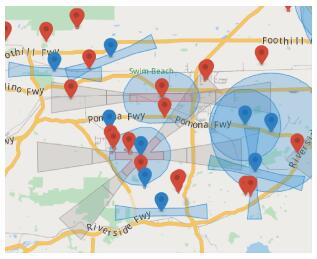图 1 限制飞行区域 Fig. 1 Restricted flight area

2.1 场景与模型描述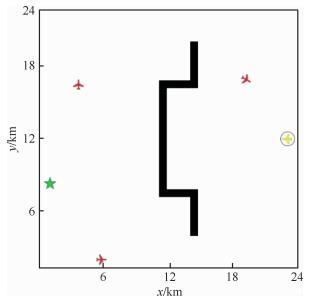图 2 仿真场景1模型示意图 Fig. 2 Model chart of simulation Scenario 1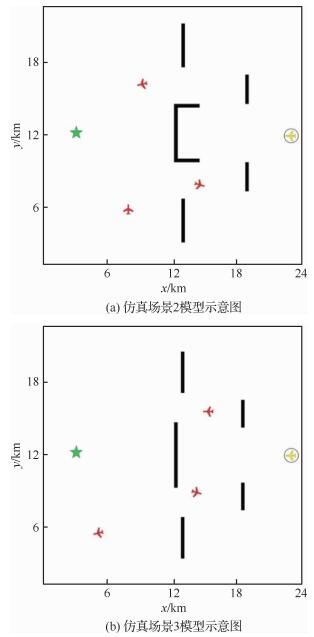图 3 限飞空域场景模型示意图 Fig. 3 Model chart of restricted airspace scenario

 $\begin{array}{l} \left\{ {\begin{array}{*{20}{l}} {{\rm{123}}}&{\Delta d \ge 555{\kern 1pt} {\kern 1pt} {\kern 1pt} {\rm{m}}}\\ {{\rm{123}}}&{150{\kern 1pt} {\kern 1pt} {\kern 1pt} {\rm{m}} \le \Delta d \le 555{\kern 1pt} {\kern 1pt} {\kern 1pt} {\rm{m}}}\\ {{\rm{123}}}&{\Delta d \le 150{\kern 1pt} {\kern 1pt} {\kern 1pt} {\rm{m}}} \end{array}} \right.\\ \Delta d = \sqrt {{{({i_x} - {o_x})}^2} + {{({i_y} - {o_y})}^2}} \end{array}$ （4）

2.2 飞行器运动模型

 $\left\{ {\begin{array}{*{20}{l}} {\varepsilon \backsim N(0, {\sigma ^2})}&{}\\ {{v^\prime } = v + {a_v} \times \Delta t + {\varepsilon _v}}&{{\varepsilon _v}\backsim N(0, {2^2})}\\ {{\varphi ^\prime } = \varphi + {a_\varphi } \times \Delta t + {\varepsilon _\varphi }}&{{\varepsilon _\varphi }\backsim N(0, {4^2})}\\ {\theta = \frac{{g{\kern 1pt} {\kern 1pt} {\rm{tan}}{\kern 1pt} {\kern 1pt} {\kern 1pt} {\varphi ^\prime }}}{{{v^\prime }}}}&{}\\ {{x^\prime } = x + v{\kern 1pt} {\kern 1pt} {\rm{cos}}{\kern 1pt} {\kern 1pt} {\kern 1pt} \theta \times \Delta t}&{{\varepsilon _x}\backsim N(0, {{10}^2})}\\ {{y^\prime } = y + v{\kern 1pt} {\kern 1pt} {\rm{sin}}{\kern 1pt} {\kern 1pt} {\kern 1pt} \theta \times \Delta t}&{{\varepsilon _y}\backsim N(0, {{10}^2})} \end{array}} \right.$ （5）

2.3 状态集描述

 ${S_{\rm{T}}} = \left\{ \begin{array}{l} {\rm{试验机飞出模型边界}}\\ {\rm{试验机发生碰撞}}\\ {\rm{试验机抵达目标点}} \end{array} \right.$ （6）

 $\begin{array}{l} ({o_x}{o_y}{o_v}{o_\theta }i_x^{(1)}i_y^{(1)}i_v^{(1)}i_\theta ^{(1)} \cdots \\ {\kern 1pt} {\kern 1pt} {\kern 1pt} {\kern 1pt} {\kern 1pt} {\kern 1pt} {\kern 1pt} {\kern 1pt} {\kern 1pt} {\kern 1pt} {\kern 1pt} {\kern 1pt} {\kern 1pt} {\kern 1pt} {\kern 1pt} {\kern 1pt} {\kern 1pt} {\kern 1pt} {\kern 1pt} {\kern 1pt} {\kern 1pt} {\kern 1pt} i_x^{(k)}i_y^{(k)}i_v^{(k)}i_\theta ^{(k)} \cdots i_x^{(n)}i_y^{(n)}i_v^{(n)}i_\theta ^{(n)}{g_x}{g_y}) \end{array}$

2.4 动作集描述

 $\left\{ {\begin{array}{*{20}{l}} {{A_{\rm{a}}} \in \{ - 5, 0, 5\} {\kern 1pt} {\kern 1pt} {\kern 1pt} {\rm{m/}}{{\rm{s}}^2}}\\ {{A_\varphi } \in \{ - 5, 0, 5\} {\kern 1pt} {\kern 1pt} {\kern 1pt} {(^\circ })/{\rm{s}}}\\ {({a_v}, {a_\varphi }) \in {A_{\rm{a}}} \times {A_\varphi }} \end{array}} \right.$ （7）

2.5 奖励函数描述

 $R(S) = \left\{ {\begin{array}{*{20}{l}} 1&{{\rm{抵达目标点}}}\\ {1 - \frac{{d(g)}}{{{\rm{max}}(d(g))}}}&{{\rm{正常飞行}}}\\ 0&{{\rm{发生碰撞}}} \end{array}} \right.$ （8）

MCTS算法需要进行大量的随机过程模拟采样以获得最佳策略，所以将其应用于无人机避撞时，考虑到对算法实时性的要求，不可能进行长时间的运算来得到全局最优解，而只能在有限时间里得到当前状态下的一个局部最优解。因此，MCTS算法仅能满足短期内的局部冲突避撞。

 $\begin{array}{l} R(S) = \\ {\kern 1pt} {\kern 1pt} {\kern 1pt} {\kern 1pt} {\kern 1pt} {\kern 1pt} {\kern 1pt} {\kern 1pt} {\kern 1pt} {\kern 1pt} {\kern 1pt} {\kern 1pt} {\kern 1pt} {\kern 1pt} \left\{ {\begin{array}{*{20}{l}} 1&{{\rm{抵达目标点}}}\\ {1 - \frac{{d(p)}}{{{\rm{max}}(d(p))}} \times a - }&{}\\ {{\kern 1pt} {\kern 1pt} {\kern 1pt} {\kern 1pt} {\kern 1pt} {\kern 1pt} {\kern 1pt} {\kern 1pt} {\kern 1pt} {\kern 1pt} {\kern 1pt} {\kern 1pt} {\kern 1pt} {\kern 1pt} {\kern 1pt} {\kern 1pt} {\kern 1pt} {\kern 1pt} {\kern 1pt} {\kern 1pt} {\kern 1pt} \frac{{d(g)}}{{{\rm{max}}(d(g))}} \times (1 - a)}&{{\rm{正常飞行}}}\\ 0&{{\rm{发生碰撞}}} \end{array}} \right. \end{array}$ （9）

2.6 算法描述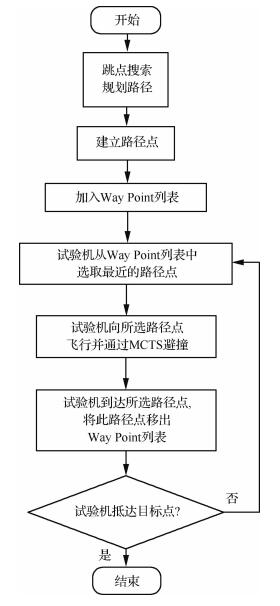图 4 算法流程示意图 Fig. 4 Flow chart of algorithm

3 仿真与结果分析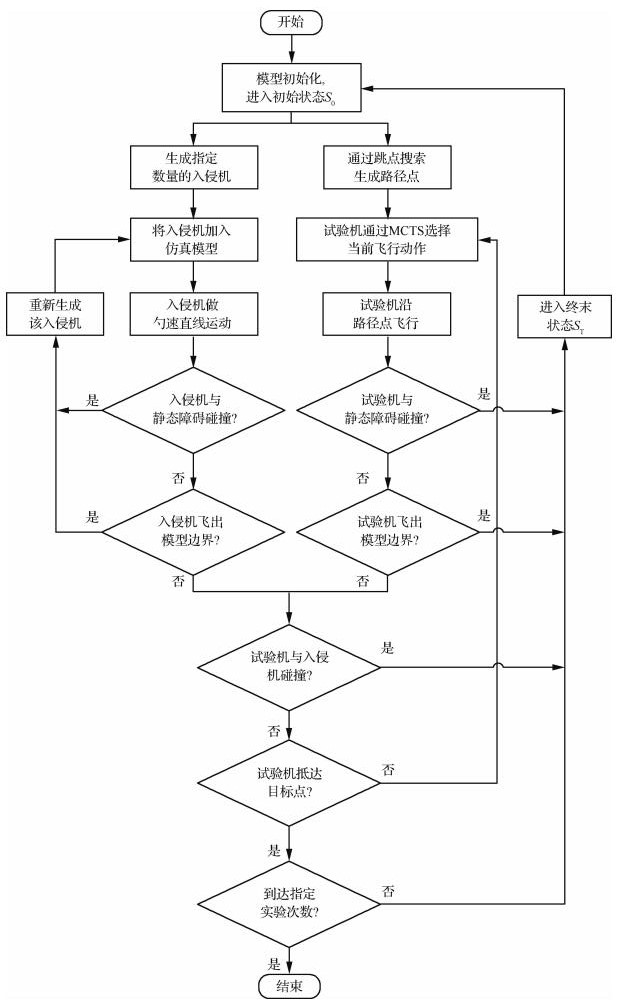图 5 仿真流程示意图 Fig. 5 Flow chart of simulation
3.1 设置实验参数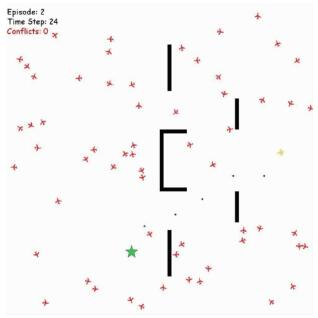图 6 仿真画面 Fig. 6 Simulation animation
 $\left\{ {\begin{array}{*{20}{l}} {{P_{\rm{n}}} = \frac{{{C_{\rm{n}}}}}{N}}\\ {{P_{\rm{c}}} = \frac{{{C_{\rm{c}}}}}{N}}\\ {{P_{\rm{g}}} = \frac{{{C_{\rm{g}}}}}{N}} \end{array}} \right.$ （10）

3.2 选取路径点的间隔距离

 路径点间隔/像素 NMAC概率 冲突概率 抵达目标点概率 平均飞行时间/s 40 0.014 0.166 0.976 469 60 0.006 0.178 0.986 452 80 0.004 0.172 0.986 453 100 0.008 0.16 0.974 437 120 0.01 0.176 0.970 445 140 0.01 0.21 0.976 417 160 0.04 0.204 0.958 434

3.3 选取奖励函数的比例系数

 比例系数 NMAC概率 冲突概率 抵达目标点概率 平均飞行时间/s 1.0 0.008 0.224 0.950 476 0.9 0.008 0.192 0.950 470 0.8 0.004 0.216 0.950 448 0.7 0.008 0.266 0.940 455 0.6 0.014 0.202 0.938 462 0.5 0.008 0.174 0.850 489 0.4 0.016 0.214 0.722 501

3.4 对比实验

 算法 NMAC概率 冲突概率 抵达目标点概率 平均飞行时间/s 平均路径长度/km MCTS 0.014 0.328 0.702 846 53 JPS-MCTS 0.004 0.210 0.984 442 30

 算法 NMAC概率 冲突概率 抵达目标点概率 平均飞行时间/s 平均路径长度/km MCTS 0.072 0.148 0.926 441 30 JPS-MCTS 0.018 0.140 0.976 371 25

 算法 NMAC概率 冲突概率 抵达目标点概率 平均飞行时间/s 平均路径长度/km MCTS 0.018 0.156 0.978 392 26 JPS-MCTS 0.016 0.148 0.984 367 25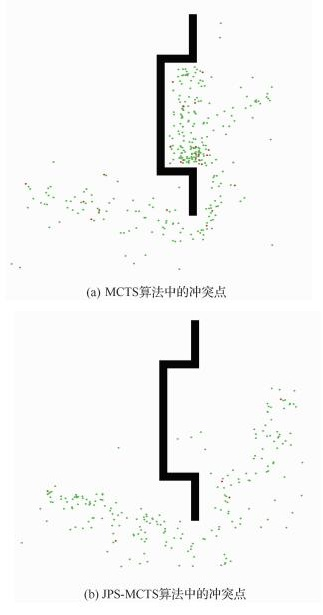图 7 场景1中冲突点对比结果 Fig. 7 Comparison of conflict positions in Scenario 1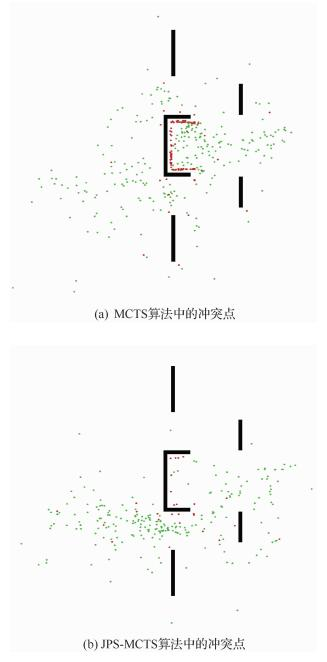图 8 场景2中冲突点对比结果 Fig. 8 Comparison of conflict positions in Scenario 2
4 结论

  THIPPHAVONG D P, APAZA R, BARMORE B, et al. Urban air mobility airspace integration concepts and considerations[C]//2018 Aviation Technology, Integration, and Operations Conference, 2018: 3676.  Uber Elevate. Fast-forwarding to a future of on-demand urban air transportation[EB/OL].(2016-10-27)[2019-12-05]. https: //d1nyezh1ys8wfo.cloudfront.net/static/PDFs/Elevate%2BWhitepaper.pdf.  BALAKRISHNAN K, POLASTRE J, MOOBERRY J, et al. Blueprint for the sky[EB/OL].[2019-12-05].https: //storage.googleapis.com/blueprint/Airbus_UTM_Blueprint.pdf.  VASCIK P D, HANSMAN R J, DUNN N S. Analysis of urban air mobility operational constraints[J]. Journal of Air Transportation, 2018, 26(4): 133-146. Click to display the text  YU X, ZHANG Y. Sense and avoid technologies with applications to unmanned aircraft systems:Review and prospects[J]. Progress in Aerospace Sciences, 2015, 74: 152-166. Click to display the text  HUNTER G, WEI P. Service-oriented separation assurance for small UAS traffic management[C]//2019 Integrated Communications, Navigation and Surveillance Conference (ICNS). Piscataway: IEEE Press, 2019: 1-11.  KUCHAR J K, YANG L C. A review of conflict detection and resolution modeling methods[J]. IEEE Transactions on Intelligent Transportation Systems, 2000, 1(4): 179-189. Click to display the text  刘慧颖, 白存儒, 杨广珺. 无人机自主防撞关键技术与应用分析[J]. 航空工程进展, 2014, 5(2): 141-147. LIU H Y, BAI C R, YANG G J. Application and analysis discussion of autonomous collison avoidance techniques for unmanned aerial vehicle[J]. Advances in Aeronautical Science and Engineering, 2014, 5(2): 141-147. (in Chinese) Cited By in Cnki | Click to display the text  朱代武. 低空空域飞行冲突避让算法[J]. 交通运输工程学报, 2005(3): 73-76. ZHU D W. Calculational methods of avoiding flight conflict in low altitude airspace[J]. Journal of Traffic and Transportation Engineering, 2005(3): 73-76. (in Chinese) Cited By in Cnki | Click to display the text  PARK J W, OH H D, TAHK M J. UAV collision avoidance based on geometric approach[C]//2008 SICE Annual Conference. Piscataway: IEEE Press, 2008: 2122-2126.  TANG J, ALAM S, LOKAN C, et al. A multi-objective approach for dynamic airspace sectorization using agent based and geometric models[J]. Transportation Research Part C:Emerging Technologies, 2012, 21(1): 89-121. Click to display the text  彭良福, 林云松. 空中自动防撞系统最优逃避机动的确定[J]. 控制理论与应用, 2010, 27(11): 1575-1579. PENG L F, LIN Y S. Determination of optimal escape maneuver for automatic air collision avoidance system[J]. Control Theory & Applications, 2010, 27(11): 1575-1579. (in Chinese) Cited By in Cnki | Click to display the text  LIU J Y, GUO Z Q, LIU S Y. The simulation of the UAV collision avoidance based on the artificial potential field method[C]//Advanced Materials Research, 2012, 591-593: 1400-1404.  SUN J, TANG J, LAO S. Collision avoidance for cooperative UAVs with optimized artificial potential field algorithm[J]. IEEE Access, 2017, 5: 18382-18390. Click to display the text  YANG Z, LI C, LIU G. Cooperative 4D guidance for multiple UAVs based on tensor field[C]//2018 13th World Congress on Intelligent Control and Automation (WCICA). Piscataway: IEEE Press, 2018: 1709-1714.  梁宵, 王宏伦, 李大伟, 等. 基于流水避石原理的无人机三维航路规划方法[J]. 航空学报, 2013, 34(7): 1670-1681. LIANG X, WANG H L, LI D W, et al. Three-dimensional path planning for unmanned aerial vehicles based on principles of stream avoiding obstacles[J]. Acta Aeronautica et Astronautica Sinica, 2013, 34(7): 1670-1681. (in Chinese) Cited By in Cnki | Click to display the text  WANG H, LYU W, YAO P, et al. Three-dimensional path planning for unmanned aerial vehicle based on interfered fluid dynamical system[J]. Chinese Journal of Aeronautics, 2015, 28(1): 229-239. Click to display the text  ALONSO-AYUSO A, ESCUDERO L F, MARTIN-CAMPO F J. Collision avoidance in air traffic management:A mixed-integer linear optimization approach[J]. IEEE Transactions on Intelligent Transportation Systems, 2011, 12(1): 47-57. Click to display the text  YANG H, ZHAO Y. Trajectory planning for autonomous aerospace vehicles amid known obstacles and conflicts[J]. Journal of Guidance, Control, and Dynamics, 2004, 27(6): 997-1008. Click to display the text  CEKMEZ U, OZSIGINAN M, SAHINGOZ O K. Multi colony ant optimization for UAV path planning with obstacle avoidance[C]//2016 International Conference on Unmanned Aircraft Systems (ICUAS). Piscataway: IEEE Press, 2016: 47-52.  SHAKHATREH H, SAWALMEH A H, AL-FUQAHA A, et al. Unmanned aerial vehicles (UAVs):A survey on civil applications and key research challenges[J]. IEEE Access, 2019, 7: 48572-48634. Click to display the text  GUPTA J K, EGOROV M, KOCHENDERFER M. Cooperative multi-agent control using deep reinforcement learning[C]//International Conference on Autonomous Agents and Multiagent Systems. Berlin: Springer, 2017: 66-83.  CHEN L, PAPANDREOU G, KOKKINOS I, et al. Deeplab:Semantic image segmentation with deep convolutional nets, atrous convolution, and fully connected crfs[J]. IEEE Transactions on Pattern Analysis and Machine Intelligence, 2017, 40(4): 834-848. Click to display the text  YANG X, DENG L, WEI P. Multi-agent autonomous on-demand free flight operations in urban air mobility[C]//AIAA Aviation 2019 Forum. Reston: AIAA, 2019: 3520.  BAI H, HSU D, KOCHENDERFER M J, et al. Unmanned aircraft collision avoidance using continuous-state POMDPs[J]. Robotics:Science and Systems Ⅶ, 2012, 1: 1-8. Click to display the text  MUELLER E R, KOCHENDERFER M. Multi-rotor aircraft collision avoidance using partially observable Markov decision processes[C]//AIAA Modeling and Simulation Technologies Conference. Reston: AIAA, 2016: 3673.  YANG X, WEI P. Autonomous on-demand free flight operations in urban air mobility using Monte Carlo tree search[C]//International Conference on Research in Air Transportation (ICRAT), 2018.  BELLMAN R. A Markovian decision process[J]. Journal of Mathematics and Mechanics, 1957, 6: 679-684. Click to display the text  KOCSIS L, SZEPESVÁRI C, WILLEMSON J. Improved Monte-Carlo search[D]. Tartu: University of Tartu, 2006. Click to display the text  KOCSIS L, SZEPESVÁRI C. Bandit based monte-carlo planning[C]//European Conference on Machine Learning. Berlin: Springer, 2006: 282-293.  AUER P, CESA-BIANCHI N, FISCHER P. Finite-time analysis of the multiarmed bandit problem[J]. Machine Learning, 2002, 47(2-3): 235-256. Click to display the text  BROWNE C B, POWLEY E, WHITEHOUSE D, et al. A survey of Monte Carlo tree search methods[J]. IEEE Transactions on Computational Intelligence and AI in Games, 2012, 4(1): 1-43. Click to display the text  HARABOR D D, GRASTIEN A. Online graph pruning for pathfinding on grid maps[C]//Twenty-Fifth AAAI Conference on Artificial Intelligence. Palo Alto: AAAI, 2011.  HART P E, NILSSON N J, RAPHAEL B. A formal basis for the heuristic determination of minimum cost paths[J]. IEEE Transactions on Systems Science and Cybernetics, 1968, 4(2): 100-107. Click to display the text  BOSSON C, LAUDERDALE T A. Simulation evaluations of an autonomous urban air mobility network management and separation service[C]//2018 Aviation Technology, Integration, and Operations Conference, 2018: 3365.  DUBINS L E. On curves of minimal length with a constraint on average curvature, and with prescribed initial and terminal positions and tangents[J]. American Journal of Mathematics, 1957, 79(3): 497-516. Click to display the text  ONG H Y, KOCHENDERFER M J. Markov decision process-based distributed conflict resolution for drone air traffic management[J]. Journal of Guidance, Control, and Dynamics, 2017, 40(1): 69-80. Click to display the text
http://dx.doi.org/10.7527/S1000-6893.2020.23726

0

#### 文章信息

LI Anti, LI Chenglong, WU Dingjie, WEI Peng

Collision avoidance decision method for UAVs in random search combined with jump point guidance

Acta Aeronautica et Astronautica Sinica, 2020, 41(8): 323726.
http://dx.doi.org/10.7527/S1000-6893.2020.23726